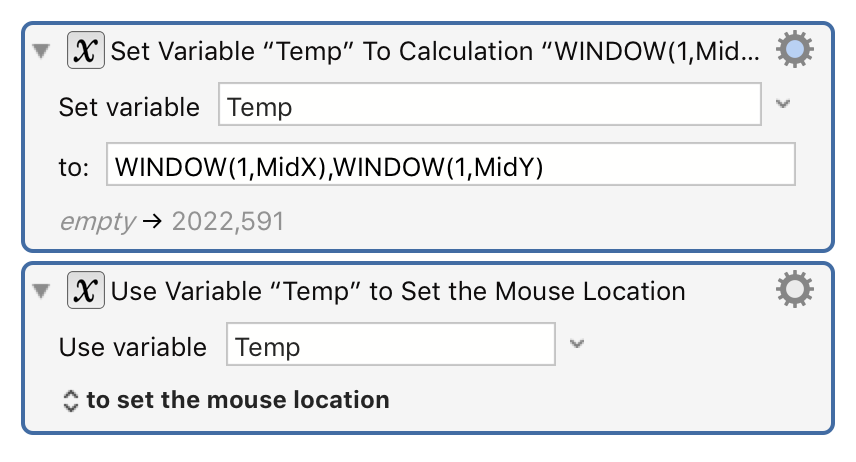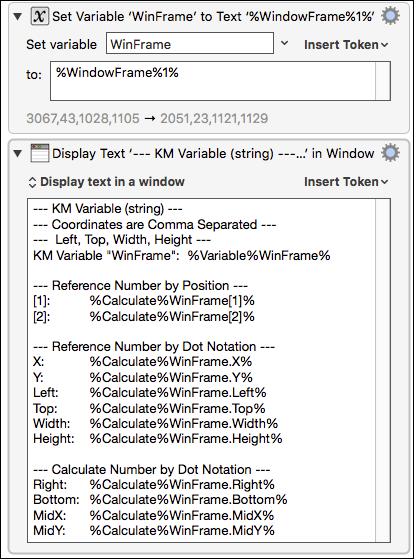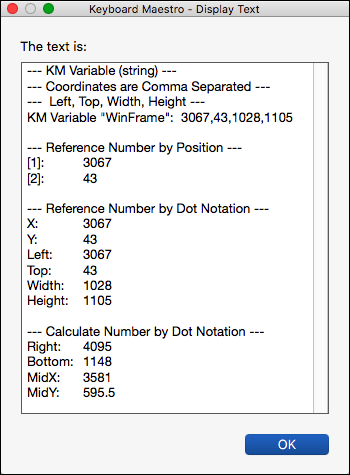#Keyboard Maestro Wiki

### Site Tools

manual:Calculations

# Calculations

## Overview

Keyboard Maestro supports calculations in almost any numeric text fields. For example you can Pause for “60*Time in Minutes”. Calculations can also use comma separated lists of numbers as arrays, and can return such arrays, so you can operate on rectangle frames and points. Numeric fields often start small with up/down step arrows, but if you type anything other than a number they will expand to allow a more complex expression to be entered. You can tell that a field accepts calculations because a small C shows in the field while editing it.

## Examples

For example:will result in the mouse being placed at the center of the front window.

Note: You must use commas for this purpose, and full stops (.) for decimal numbers, and never use any thousands separators, regardless of your desired language.

## Expressions

Keyboard Maestro’s expressions include precedence, nested bracketed expressions, many built-in functions, various numeric bases, so you should be able to write almost any expressions you might like to use, as well as use it as a general purpose calculator if desired.

## Operators

Operators based on precedence from lowest to highest are:

 Array Separator (`,`) Allows a text Variable, which has comma separated values, to work somewhat like an Array. Assume `MyKMVariable` contains “value1,value2,value3”, then `MyKMVariable` returns “value2”. Ternary Operator (`?`) a=b ? 3 : 4 Bitwise OR (`|`), Bitwise AND (`&`) and Bitwise XOR bitwise operators, which also act as logical boolean operators for 0 (false) and 1 (true). Comparison Operators (`<, ≤, =, >, ≥, ≠`) compare for (in)equality and return 0 or 1. Shift Operators (`≪, ≫`) shift a number left or right. Arithmetic Addition Operators   • Add (`+`)   • Subtract (`-`) Basic mathematical operations. Arithmetic Multiplication Operators   • Multiply (`*`)   • Divide (`/`) Basic mathematical operations. Modulo (`i MOD n`) The remainder of the division of `i` by `n`. Both values (`i`, `n`) will be treated as integer. `n` must not be 0. the sign of the result is the same as the sign of i. Integer division (`i DIV n`) The integer quotient of the division of `i` by `n`. Both values (`i`, `n`) will be treated as integer. `n` must not be 0. the sign of the result matches the sign of regular division. Power Operator (`^`) exponentiation. Unary Prefix Operators (`√`, `-`, `( )` ) square root, negation, sub-expressions. Functions a large variety of functions. Numbers and Variables or Array Accesses (5,\$5A,0x50,8#007,Variable,Variable) identifiers and values. Unary Postfix Operators (`!,%,°`)) factorial, percent, degrees.

Numbers are in decimal by default, but may use base 16 if they start with `\$` or `0x` (eg \$5A or 0x5A), or may start with a specific base followed by a `#` (eg 8#007). Numbers may include underscores, for example 53_000_000.

Variables can be used if they contain numeric expressions, including an array of numbers separated by commas, in which case you can use an array index to select the desired number.

You can use either `=` or `==` for testing for equality.

Operators and functions must be in uppercase to minimize conflict with variables.

## Functions

The available functions are listed on the wiki Functions page.

You can insert a function by name by choosing from the Edit ➤ Insert Function menu or choosing the Edit ➤ Insert Function by Name menu.

## Variables

In numeric calculation fields, Variable Names are used without the `%` that are used in text token fields. Do not try to use tokens (like `%Variable%MyVar%`) in numeric calculation fields, just use MyVar by itself. The variable must contain a valid numeric value, or an expression that evaluates to a valid numeric value. So for example, if MyVar contains a text value of `2*3`, then the calculation `4*MyVar` will return 24.

## Screen Coordinates

Keyboard Maestro refers to screen coordinates as two or four comma separated numbers in the text of a Keyboard Maestro Variable (which is always a string).

• Screen object points, like the left,top position of a window, have two values, like 12,34
• Screen object rectangles, like the frame of a window, have four values 12,34,56,78 (with a fifth value for fuzz in some instances).
* You can reference these values by position in the string, as it the Variable were an array:
• For example:
if the Variable `myWindow` is “12,34,56,78”
then all of these forms of reference will provide a value of 34 in a Calculation field:
• myWindow
• myWindow.y
• myWindow.Top

## Variable Array Access

If a variable contains a sequence of numbers separated by comma (,) then you can access that variable using array notation (eg `MyVar`). So if variable MyVar has a text value of `10,20,30,40,50,60`, MyVar will return 50.

The index is itself an expression, so it can be arbitrarily complex.

Indices are 1-based, so MyVar is the first element. If the index is 0, the size of the array is returned (so MyVar would be `6`). If the index is less than zero, the array is indexed from the end (so MyVar[-5] would be `20`).

## Variable Dot Notation

In a Calculation field you can reference the numbers in a Keyboard Maestro Variable (which is always a string) using dot notation:

 Variable.x x coordinate Variable.y y coordinate Variable.left the left coordinate of a rectangle Variable.top the top coordinate of a rectangle Variable.right the right coordinate of a rectangle Variable.bottom the right coordinate of a rectangle Variable.width the width of a rectangle or size Variable.height the height of a rectangle or size Variable.fuzz the fuzz of an image match (rectangle,fuzz) Variable.MidX the horizontal middle of a rectangle Variable.MidY the vertical middle of a rectangle

The Variable Name and Dot reference are case insensitive.

## Text Fields

In calculation fields, you can express a calculation as you would normally write an expression, for example:

`3 * Count + 7`

However in a text field, since any text is allowed, you must use percent encoded tokens to indicate where more processing is required. You can include a variable in the text by using the %Variable% token, or you can use a calculation by using the %Calculate% token, or any number of other Tokens.

`The result is %Calculate%3 * Count + 7%.`

## Examples of Variable Data Reference

Macro ActionsExample ResultsSome example expressions are:

```Amount in Dollars * 100
MJD() > 55928
NOW() > TIME(2012,3,23,12,2,1)
DOW(TIME(2012,4,4)) = 4